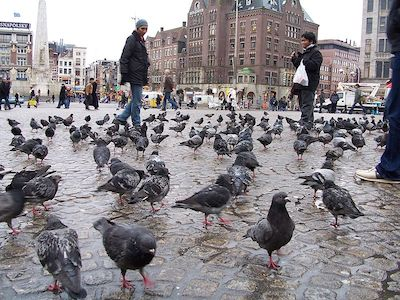# All animals are equal, but some animals are more equal than others

Probability Level 2There are $51$ people in a room. What is the largest value of $n$ such that the statement "At least $n$ people in this room have birthdays falling in the same month" is always true?

Details and Assumptions

• Assume the probability of each person's birthday is random and independent from one another.
×